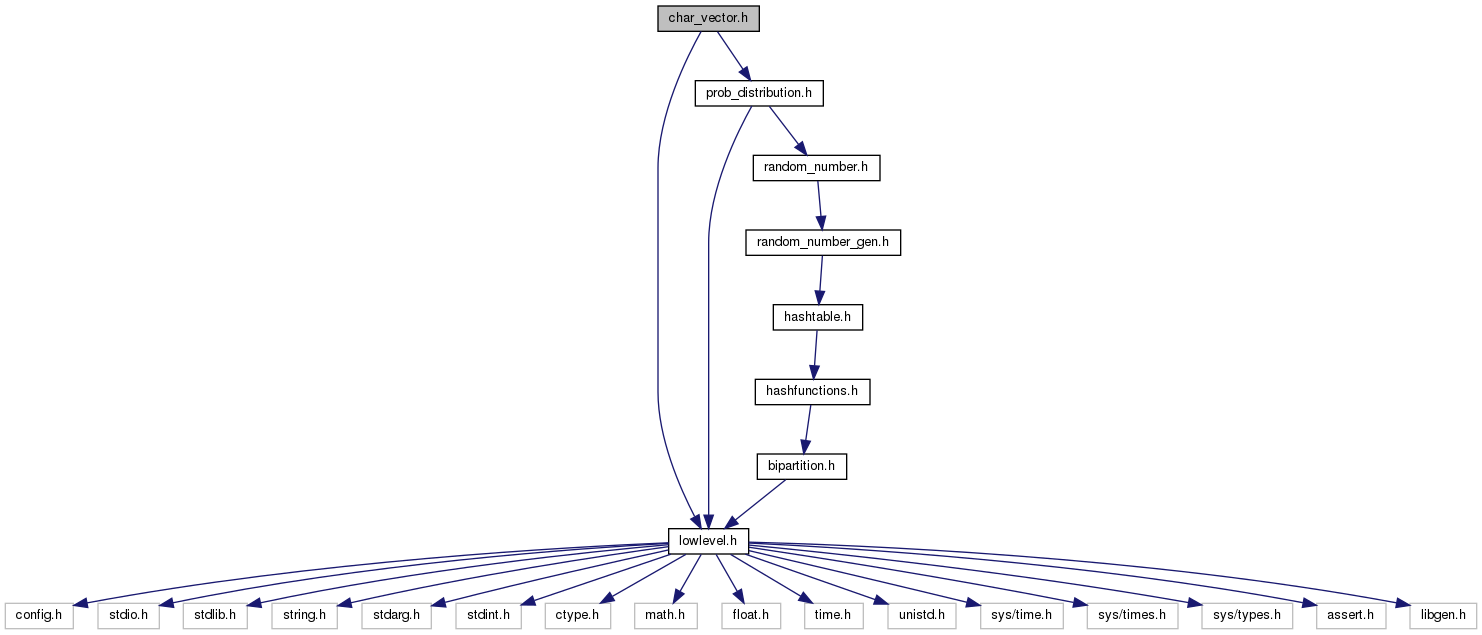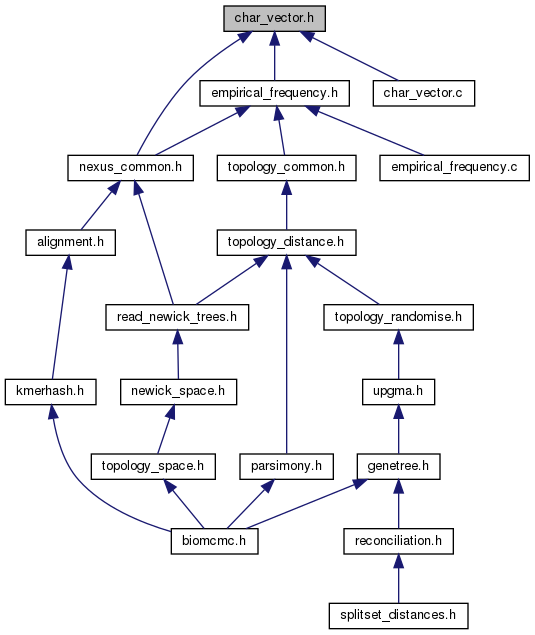biomcmc-lib  0.1 low level library for phylogenetic analysis
char_vector.h File Reference

list of strings (each string is a vector of chars) More...

`#include "lowlevel.h"`
`#include "prob_distribution.h"`
Include dependency graph for char_vector.h:This graph shows which files directly or indirectly include this file:Go to the source code of this file.

## Data Structures

struct  char_vector_struct
vector of strings (char vectors) of variable length More...

## Typedefs

typedef struct char_vector_structchar_vector

## Functions

char_vector new_char_vector (int nstrings)
Create a vector of strings with initial size for each string of zero.

char_vector new_char_vector_big (int nstrings)
Create vector of strings, and preparing it to realloc() fewer times; used in conjunction with 'append_big'.

char_vector new_char_vector_from_valid_strings_char_vector (char_vector vec, int *valid, int n_valid)
Create a vector of strings from subset of strings of another char_vector.

char_vector new_char_vector_fixed_length (int nstrings, int nchars)
Create a vector of strings where each string is assigned an initial value of nchars.

void del_char_vector (char_vector vec)
Delete vector of strings only after nobody is using it.

void char_vector_link_string_at_position (char_vector vec, const char *string, int position)
Link a previously allocated string (to avoid copying all characters)

void char_vector_add_string_at_position (char_vector vec, const char *string, int position)
Add a new string (vector of characters) at specific location.

void char_vector_add_string (char_vector vec, const char *string)
Add a new string (vector of characters) at next available location.

void char_vector_append_string_at_position (char_vector vec, const char *string, int position)
Append string at the end of existing string at location.

void char_vector_append_string (char_vector vec, const char *string)
Append string at the end of existing string at most recently used location.

void char_vector_append_string_big_at_position (char_vector vec, const char *string, int position)
Append strings like before, but doubling allocation space if insufficient (reduces calls to realloc() )

void char_vector_append_string_big (char_vector vec, const char *string)

void char_vector_finalise_big (char_vector vec)

void char_vector_expand_nstrings (char_vector vec, int new_size)
Increase size of vector of strings (called automatically by other functions)

void char_vector_reorder_strings_from_external_order (char_vector vec, int *order)
update order of strings in vector based on a vector of new positions

int char_vector_remove_empty_strings (char_vector vec)
Reduce size of vector of strings by removing empty strings (returns number of empty strings)

int char_vector_remove_duplicate_strings (char_vector vec)
Remove identical strings and resizes char_vector_struct.

void char_vector_reduce_to_valid_strings (char_vector vec, int *valid, int n_valid)
reduce char_string_struct to only those elements indexed by valid[]

void char_vector_reorder_by_size_or_lexicographically (char_vector vec, bool lexico, int *order)
Order char_vector_struct elements from longer string to smaller, or lexicographically; can be used after calling new_char_vector_from_file() but if topology etc. are associated to it, then order[] must be externally defined and will have new locations, to keep track of changes.

If the two char_vectors are identical (same strings in same order), then delete one and make it point to the other one.

void index_species_gene_char_vectors (char_vector species, char_vector gene, int *sp_idx_in_gene, int *order_external)
find occurences of species->string[] inside gene->string[] filling indexes in sp_idx_in_gene. More...

void update_species_count_from_gene_char_vector (char_vector species, char_vector gene, int *sp_count)

## Detailed Description

list of strings (each string is a vector of chars)

## ◆ index_species_gene_char_vectors()

 void index_species_gene_char_vectors ( char_vector species, char_vector gene, int * sp_idx_in_gene, int * order_external )

find occurences of species->string[] inside gene->string[] filling indexes in sp_idx_in_gene.

The species are taxon names which may be associated with topologies or alignments, such that we can not reorder its elements here (without also modifing e.g. tree leaves). But ordering from longer to shorter is essential for pattern finding, so it is assumed that the char_vector is already sorted UNLESS user provides the ordering.